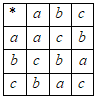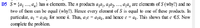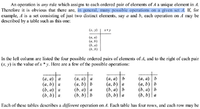# Subgroups

#### bZNyQ7C2

##### New member
I'm working my way through A Book of Abstract Algebra by Charles Pinter. I ran into a snag on page 54. This is problem C5:
"Let G be a finite group and let S be a nonempty subset of G. Suppose S is closed with respect to multiplications. Prove that S is a subgroup of . (Hint: it remains to prove that S contains e and is closed with respect to inverses. Let $$\displaystyle S = \left\{ {{a_1}, \ldots {a_n}} \right\}$$. If $$\displaystyle {a_i} \in S$$, consider the distinct elements $$\displaystyle {a_i}{a_1},{a_i}{a_2}, \ldots {a_i}{a_n}$$.)"

OK ... I did figure out part of it. If I imagine a Cayley Table for S, the set of elements $$\displaystyle {a_i}{a_1},{a_i}{a_2}, \ldots {a_i}{a_n}$$ will be a row in it. That row will be a selection from the corresponding row of the Cayley Table for G. No row in the Cayley Table for G has any duplicates, so no selection from it can have any duplicates either. If S is closed under multiplication, then $$\displaystyle {a_i} \in S$$, then $$\displaystyle {a_i}$$ must appear in that row somewhere. For some k, $$\displaystyle {a_i} = {a_i}{a_k}$$ and then $$\displaystyle e ={a_k}$$.

Or does it? Actually, I don't think so. I think that last step fails.

Here's a sample Cayley Table, not a group, but a binary structure on the set $$\displaystyle \left\{ {a,b,c} \right\}$$:Every element appears in every row and column, nothing appears in any cell that isn't an element of the set, but there's no identity.

Either I disproved what Dr. PInter wants me to prove, or I made a mistake. Can anybody show me where I went wrong?

#### Cubist

##### Full Member
It's been years since I've done group theory, but since no-one else has replied yet I thought I'd give my input. I think your point is valid. The question does seem to hint towards the solution that you give. And I think you're correct that it alone isn't enough to prove that there is an element e. Well spotted!

However the question does mention that S is closed under multiplication. Perhaps if this is taken into account during the proof somehow it might force the element "1" to be in the group, which would obviously be e. ( It feels kind of wrong to say that e is 1)

EDIT:
For some k, $$\displaystyle {a_i} = {a_i}{a_k}$$ and then $$\displaystyle e ={a_k}$$.
...actually the above line in your proof forces e to be 1, since some $$a_i$$ must be non zero? And I think that your specific Cayley Table could not be generated under multiplication if "1" is an element?

Last edited:

#### bZNyQ7C2

##### New member
I actually spotted my mistake shortly I made the post.

The example table I gave is possible if we start from scratch, but we're not. We're given the S is a subset of G and the operation is the same as G. The table for S must contain only entries that also occur in the table for G. In the table for G, all the entries that return a for a or b for b etc would involve multiplying a or b by e.

Seems very simple once you see it. I feel dumb for not having seen it earlier, a familiar feeling to me.

#### pka

##### Elite Member
You posted For some $$k, a_i=a_ia_ka_i=a_ia_k$$ and then $$e=a_ke=a_k.$$ I don't think that follows at all.
Here is a theorem I would try. The ssubset $$S$$ of a group $$G$$ is a subgroup if and only if $$\forall \{a,b\}\subset S$$ it must be true that $$ab^{-1}\in S$$.

#### bZNyQ7C2

##### New member
@pka ... We are given that S is a subset of G and also that S is closed under multiplication. From the context, I gather that the operation on S and the operation on G are the same.

Given those two things, I believe it does follow. I didn't see it at first, but now I'm convinced.

Why do you think it does not follow?

#### pka

##### Elite Member
Please give a coherent argument then. So far I see hand waving.

#### bZNyQ7C2

##### New member
Well, Dr. Pinter kindly provided back-of-the-book remarks on some of these problems. Here's what he said about this problem on page 332 ...I should've mentioned that I adapted his explanation, and that last part about "for some k" I quoted directly. I should've explicitly mentioned the quote.

I never doubted that it was true what he asked me to prove, nor that his explanation was correct. I figured I was missing something, but I couldn't figure out what. Now I think I know.

I will explain it in the way that makes best sense to me. If you have a better way, I'd be grateful if you shared it with me.

We're given that S is a subset of G, that is, every element of S is also in G, and that S is closed under multiplication. By "multiplication," I assume he means whatever operation is defined on the group G. That operation must have an identity e, because G is a group. I'll denote that operation *. When we combine two elements $$\displaystyle {a_3},{a_k}$$ of G using that operation, then there is only one way to get $$\displaystyle {a_3}*{a_k} = {a_3}$$, and that is if $$\displaystyle {a_k} = e$$.

It helps me to think of the Cayley tables of G and S. Suppose there are n entries in S, so $$\displaystyle S = \left\{ {{a_1}, \ldots {a_n}} \right\}$$. We also know S is closed under *. In the table for S, there will be one row that contains all the products $$\displaystyle {a_i}{a_1},{a_i}{a_2}, \ldots {a_i}{a_n}$$. There are n of these products, and there are exactly n elements in S with no duplicates, so $$\displaystyle {a_i}$$ must be one, and only one, of those n products. Which one?

Remember that the table for S contain only entries that also occur in the table for G. In the table for G, there is only one entry that will give $$\displaystyle {a_i}$$ back, and that's the one in the column with an e on the top, $$\displaystyle {a_i}*e$$.

Suppose it wasn't $$\displaystyle {a_i}*e$$ but some other product $$\displaystyle {a_i}*{a_k}$$. That would mean that the Cayley table for G would contain one entry for $$\displaystyle {a_i}*e={a_i}$$ and another, duplicate entry $$\displaystyle {a_i}*{a_k}={a_i}$$. No group can have a Cayley Table with duplicate entries in the same row. Therefore, $$\displaystyle {a_k} = e$$, so $$\displaystyle e \in S$$.

Is that clear?

I think I understand it OK, but I'm still learning how to explain it.

#### topsquark

##### Full Member
Here's another objection. It isn't associative. (ab)c = cc = c. a(bc) = aa = a. A group may not be commutative, but it's always associative.

-Dan

#### Cubist

##### Full Member
By "multiplication," I assume he means whatever operation is defined on the group G.
I personally think he literally means multiplication. Can't the elements of G be the complex Nth roots of unity. This might be what Dr. Pinter is hinting at in the second sentence of the image in post#7.

For example, if G is formed from the 10th roots of unity, can't the elements of S be the 5th roots?

#### bZNyQ7C2

##### New member
@topsquark ... I think you're referring to the little 3 x 3 table I put into the original post. You're quite right, that is not the Cayley table for any group. I described it as a binary structure, not a group.

You know a lot more about math than I do, but I wonder if you're familiar with the term "binary structure." This term doesn't appear in most books, including the one I'm working from (Pinter covers the concept, but doesn't call it by that name). It's more general than "group." Informally, it means any possible arrangement of the elements of a set into a square table. For a finite group with $$\displaystyle n$$ elements, there are $$\displaystyle {n^{2n}}$$ binary structures. On a 3-element set, there are 216 binary structures, but up to isomorphism, only one group.

At that point in my thinking, I wasn't sure whether S was a group or not. That was part of what of what I was asked to prove. I thought possibly it might not be.

The table I showed does describe an operation on the set $$\displaystyle \left\{ {a,b,c} \right\}$$, and that set could be a subset of something like $$\displaystyle \left\{ {a,b,c,d,e,} \right\}$$, and on that larger set, a group does exist (there is one group of order 5, cyclic). So I thought I might've found a counterexample. I was wrong.

I didn't fully understand what it means to say S is a subset of G, when G is a group. It means that the cells in the table for S must be a subset of the Cayley Table for G. And in that Cayley Table, Pinter's argument works. Here's a general statement: a table that doesn't represent a group cannot be a subset of any larger table that does.

I'm sure you understand this. I'm saying this for the benefit of myself, and anyone else who might read this.

#### bZNyQ7C2

##### New member
@Cubist ... Your example is interesting. A finite group with an operation that looks like ordinary multiplication.

Here's what Pinter says about operations:He's being as abstract as possible (except he says "rule" instead of "mapping," as I would've done). I think he's right to do that. Notice those four funny tables at the bottom. They aren't Cayley Tables, but they do have one important advantage: they make it clear that an operation is a mapping from a set of ordered pairs to a set of single elements. Some operations on some sets produce a group. Most don't.

I think of it by analogy to sets. Consider the set $$\displaystyle \left\{ {1,3} \right\}$$. If I say, "it's the set containing the number one and the number 3," that's already a full and complete description, sticking to the facts. If I say, "it's the set of roots to a certain quadratic equation," or "it's the set of the first two odd integers," or "it's the set of the first two integers whose English names contain the letter e," then I'm going beyond facts into interpretation.

So if you made a Cayley Table describing your operation built on the 10th roots of unity, I'd say "that table is the operation ... nothing more needs to be said. If you call it multiplication, that's your interpretation. Maybe some other interpretation is possible."

.

•topsquark

#### Cubist

##### Full Member
I understand the abstract nature of group theory, both for the operator and for the elements.

I only got the idea that the operator is multiplication based on the original question:-
Suppose S is closed with respect to multiplications.
But perhaps this word means something else in this context?

Are you saying that groups G and S have an unknown operator, however the elements of S are ALSO closed under the (possibly different) operator of multiplication? This seems unlikely to me, and if this is true then the question doesn't state that S is closed under the original operator of G. That would surely throw a large spanner into any possible answer? But then again maybe the question just wasn't very well written.

#### Cubist

##### Full Member
I understand the abstract nature of group theory, both for the operator and for the elements.
I've just thought of a different type of group element that could work under multiplication:- 2x2 rotation matrices could be made to form such a group. Therefore I was wrong to quote a specific element type of "complex number" in post#9 because the element types are actually unknown. Interesting! I'll go take a nap in the corner for a while#### firemath

##### Full Member
I've just thought of a different type of group element that could work under multiplication:- 2x2 rotation matrices could be made to form such a group. Therefore I was wrong to quote a specific element type of "complex number" in post#9 because the element types are actually unknown. Interesting! I'll go take a nap in the corner for a whileSay hi to Jomo for me.

•Cubist

#### bZNyQ7C2

##### New member
Are you saying that groups G and S have an unknown operator, however the elements of S are ALSO closed under the (possibly different) operator of multiplication? This seems unlikely to me, and if this is true then the question doesn't state that S is closed under the original operator of G. That would surely throw a large spanner into any possible answer? But then again maybe the question just wasn't very well written.
Did I write "multiplications" in the plural? That was a typo I'm sure. There is only one operation here.

It's true that the problem statement doesn't exactly say that the operation of S is the same as the operation of G. But what other operation would it be? If it's any other operation, there is no question of S being a subgroup of G. It's part of the definition of a subgroup that it must have the same operation as its parent group.

If we assume that G and S have the same operation, the proof is easy. If we allow any possibility that S has a different operation ... I don't think any proof is possible. In fact, I strongly suspect that the statement offered for proof would then be simply untrue.

Was the question well written? Maybe not. The solution that Dr PInter offers uses the left cancellation property, which applies only to group operations. It's assuming what it seeks to prove. A good answer to this question, I believe, would have to avoid using that.

Yes, the rotation group is interesting.